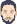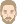## surface transfer coefficient

Everything that didn't fit in any other topic
Amandine Piot
WUFI International Support TeamPosts: 40
Joined: Mon Feb 28, 2011 11:02 pm -1100

### surface transfer coefficient

Hello dear Wufi-team,

hope you're fine.
I have the following 2 questions concerning some "internal" calculations of wufi (currently using wufi-pro 6.5):

* calculation of the surface exchange coefficients : I'm trying to compare the results of calculation of wufi with another model, and therefore we need to have the same surface exchange coefficients. On the exterior side, there is the possibility to distinguish between convection and radiation, for example by using the option "wind-dependent". I forced a value at alpha=alpha_conv=8W/m²K, alpha_rad=0, and wind coefficients =0 . When I plot the "heat tranfer coefficient", I get indeed 8W/m²K. So far so good.
For the moisture exchange coefficient: the help gives the following formula: bêta = 7e-9 * alpha_conv ; which leads to a value of 5.6e-8kg/m²sPa. Now, when I plot the "vapor transfer coefficient", I get a varying value... (see attached picture). The coefficient varies around the expected value, but with daily and yearly variations.
Could you detail how this value is calculated ? (In this particular case, there is no radiation exchanges, and no rain).

*sorption curve: the values of the sorption curve are given as a table; are the values in-between linearly interpolated ?

Thanck you very much in advance...AttachmentsCapture d’écran 2021-11-25 102112.png (14.04 KiB) Viewed 60 times

ThomasPosts: 337
Joined: Sun Jun 19, 2005 10:33 pm -1100

### Re: surface transfer coefficient

Amandine Piot wrote:
Wed Nov 24, 2021 10:33 pm -1100
Now, when I plot the "vapor transfer coefficient", I get a varying value... (see attached picture). The coefficient varies around the expected value, but with daily and yearly variations.
Could you detail how this value is calculated ? (In this particular case, there is no radiation exchanges, and no rain).
Hi Amandine,

nice to hear from you. Yes, there is a little quirk in the calculation of the $$\beta$$.

In WUFI there is for each surface the option for the user to specify an "sd-value" which describes the diffusion resistance of surface coatings like wallpapers, paint coats etc. without the need to explicitly add these as layers to the assembly.

In general, the diffusion resistance at a surface is therefore the sum of the resistance caused by the stagnant air layer adhering to the surface (always present and described by the $$\beta$$) plus the user-defined sd-value (if such an sd-value has been specified). For calculating the sum of the two resistances, the coefficient $$\beta$$ must be converted into the corresponding resistance, the conversion factor is the water vapour diffusion coefficient in air, $$\delta_a$$.

The resulting total sd-value is computed once and for all during the initialization of the simulation run:

$$s_{d, total} = s_{d, user} + \frac{\delta_a(10^\circ \mathrm{C})}{\beta}$$

At this point, the diffusion coefficient $$\delta_a$$ is evaluated for a temperature of 10°C because no simulation results are yet available.

During each step of the simulation, the current coefficients for the transport equations must be computed, and therefore the $$s_{d, total}$$ (which corresponds to a resistance) must be converted back to a coefficient:

$$\beta^\prime = \frac{\delta_a(\vartheta_a)}{s_{d, total}} = \frac{\delta_a(\vartheta_a)}{ s_{d, user} + \frac{\delta_a(10^\circ \mathrm{C})}{\beta} }$$

where the diffusion coefficient $$\delta_a$$ is now evaluated for the current air temperature $$\vartheta_a$$ at the surface in question. If the user has not specified an sd-value for that surface ($$s_{d, user} = 0$$), we have simply

$$\beta^\prime = \frac{\delta_a(\vartheta_a)}{\delta_a(10^\circ \mathrm{C})} \cdot \beta$$

For this reason the surface transfer coefficient $$\beta^\prime$$ used by WUFI depends on the air temperature. For example, we have

$$\delta_a(10^\circ \mathrm{C}) = 1.8816943 \cdot 10^{-10} \ \mathrm{kg ⁄(m s Pa)}$$ and
$$\delta_a(20^\circ \mathrm{C}) = 1.9353453 \cdot 10^{-10} \ \mathrm{kg ⁄(m s Pa)}$$

so that for an air temperature of 20 °C we have

$$\beta^\prime = \frac{\delta_a(20^\circ \mathrm{C})}{\delta_a(10^\circ \mathrm{C})} \cdot \beta = 1.0285121 \cdot \beta$$

WUFI uses the formula

$$\delta_a(\vartheta, P) = 1.968 \cdot 10^{-7} \cdot (\vartheta + 273.15)^{0.81} \cdot \frac{1}{P}$$

where $$\vartheta$$ is the temperature in °C and $$P$$ is the barometric pressure in Pa. See WUFI's help file for how WUFI determines the pressure. If no weather file with pressure data is used and the location height is not known, WUFI uses the default value 101300 Pa.

The vapour exchange coefficient at the surface is then

$$\beta^\prime = \frac{\delta_a(\vartheta_a)}{\delta_a(10^\circ \mathrm{C})} \ \cdot \ 7\cdot10^{-9} \ \cdot \ \alpha_{conv}$$

If you wish to force a certain constant $$\alpha_{conv}$$ at the exterior surface, please note that if the explicit radiation balance is switched off and wind-dependence of the exterior $$\alpha$$ is also switched off, WUFI will simply use the entered heat transfer coefficient as a constant number, and it will assume by default that the radiative part of it is 6.5 W/(m²K) (4.5 on the interior surface).

The convective part is then determined as follows:

exterior side: $$\alpha_{conv, ext} = \alpha_{ext} - 6.5 \ \mathrm{W/m²K}$$
interior side: $$\alpha_{conv, int} = \alpha_{int} - 4.5 \ \mathrm{W/m²K}$$

then the $$\beta$$ are calculated:

exterior side: $$\beta_e = 7\cdot10^{-9} \ \cdot \ \min(3.5, \alpha_{conv, ext})$$
interior side: $$\beta_i = 7\cdot10^{-9} \ \cdot \ \min(3.5, \alpha_{conv, int})$$

and finally, due to the way it has been programmed, the factor described above appears:

exterior side: $$\beta^\prime_e = \frac{\delta_a(\vartheta_a)}{\delta_a(10^\circ)} \cdot \beta_e$$
interior side: $$\beta^\prime_i = \frac{\delta_a(\vartheta_a)}{\delta_a(10^\circ)} \cdot \beta_i$$

- - - - -

To give an example: Open WUFI's example project "Example_CSTB.w6p". Switch from display of "heat transfer resistance" to "heat transfer coefficient":

Options -> Unit System -> Heat Transfer, mark "Coefficient"

Explicit radiation balance and wind-dependence of the heat transfer coefficient are off, and the "Surface Transfer" dialog shows that WUFI uses the heat transfer coefficient for the "External Wall", 17 W/(m²K).

For simple temperature conditions, set the exterior climate to a constant temperature:

Climate -> Outdoor -> Sine Curves -> User-Defined -> Temperature 10 °C, constant

Run the calculation for a few time steps, then use the ASCII-export to export the heat and vapor transfer coefficients at the exterior surface. For the $$\beta$$ we expect

$$\beta^\prime = \frac{\delta_a(10^\circ \mathrm{C})}{\delta_a(10^\circ \mathrm{C})} \ \cdot \ 7\cdot10^{-9} \ \cdot (17 - 6.5) = 7.35 \cdot 10^{-8} \ \mathrm{kg/(m² s Pa)}$$,

and that is what WUFI gives us.

Now change the exterior temperature to 20 °C, we expect

$$\beta^\prime = \frac{\delta_a(20^\circ \mathrm{C})}{\delta_a(10^\circ \mathrm{C})} \cdot \beta = 1.0285121 \cdot 7\cdot10^{-9} \ \cdot (17 - 6.5) = 7.5595639 \cdot 10^{-8} \ \mathrm{kg/(m² s Pa)}$$,

the WUFI output gives us $$7.5595636 \cdot 10^{-8} \ \mathrm{kg/(m² s Pa)}$$*sorption curve: the values of the sorption curve are given as a table; are the values in-between linearly interpolated ?
Here the answer is much shorter: yes.

Regards,
Thomas

Amandine Piot
WUFI International Support TeamPosts: 40
Joined: Mon Feb 28, 2011 11:02 pm -1100

### Re: surface transfer coefficient

hi Thomas,

thank you very much for this clear and detailed answer. (Thank you for the clear and short answer as well).